Help

# Calculate

## What is the calculate component for? #

The calculate component is intended to perform calculations on values taken from responses to other components within the same form. It contains an advanced calculation builder that enables you to create your own expression as a tree based structure.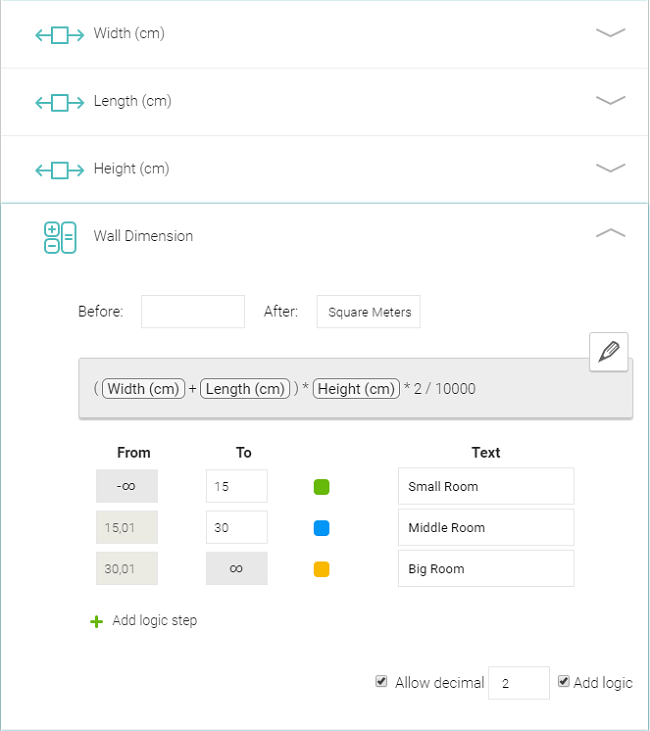## Functions and operations #

There are plenty of functions and mathematical operations you can use to create expressions using the calculation builder. You can find out how they are used in the list below:

abs: The abs function computes the absolute value of a number, which means the non-negative value of a number regardless of its sign.
It accepts exactly one parameter with a numeric value which can be taken from the following form field types: Dropdown, Single answer, Multiple answers, Number, Calculate or can be the result of an operation or function that returns a numeric value.

percentage: The percentage function calculates the percentage score achieved from the highest possible defined value. The result is computed as the sum of scores achieved for every parameter divided by the sum of the highest possible defined values of all parameters and then multiplied by 100. It accepts 1 or more parameters that can be taken from the following form field types: Dropdown, Single answer, Multiple answers or Number.

distance: The distance function computes the length of the shortest possible path through space, between two points, that can be completed if there were no obstacles. It accepts exactly two parameters of the GPS component type.

distanceTravel: The distanceTravel function computes the length of a specific path travelled between two points, such as the distance walked while navigating a maze. It accepts exactly two parameters of the GPS component type. This function is not available in the mobile app and therefore cannot be used for number logic.

timediff: The timediff function calculates the difference between two time or datetime values. It accepts exactly two parameters of either Time or DateTime field types. Parameter can be the Date & Time component with Time or Date and Time type (Date type cannot be used). There are also two special parameters Created in App and Modified from App that can be used. The result is computed as parameter2 - parameter1.
When the timediff is the root function/operation, then the result will be displayed in the format of hours:minutes (eg “8:45”). However when the timediff is used within a calculation, the result is in the number of seconds format (eg “timediff(StartTime, EndTime) / 3600” will then display a decimal number like 8.75).

+ (plus, addition): The + operation computes the total amount of two or more numeric values.

- (minus, subtraction): The - operation computes the difference between two numeric values.

* (multiplication): The * operation computes the multiplication of two or more numeric values.

/ (division):  The / operation computes the quotient of two numeric values.

^ (exponentiation): The ^ operation computes the exponentiation of two numeric values. The first argument is the base and the second argument is the exponent or power. eg: 2^3=8

( (parenthesis): the ( Used to group things together to prioritise the evaluation of parts of the expression.

If you want to use the single answer, multiple answer or dropdown component - You need to assign the values to each response first (click to Set Values button and fill the number beside the option). For example you can assign +10 to Yes and 0 to the No answer.

## How to use the calculation builder #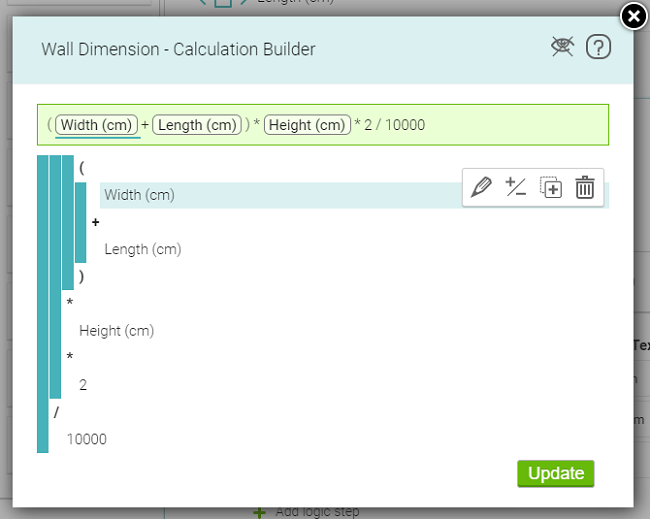To create or edit an item inside the builder tree, there is an input field with whispering functionality. When you start to type into input field, a list of suggestions containing items filtered based on your typed text is displayed. Then you can click one of them to select it. If you want to type only a numeric value, type it inside the input field and simply confirm by pressing the Enter key.

When you hover over the item inside the builder tree, the action icons are displayed on the right side of this item. These actions are:

## Edit #When you click the Edit button, the item switches to editing mode and the input field is displayed. Now you can start typing and then select a new item value from the suggestion list which again filters based on typed text, or confirm the numeric value by pressing the Enter key.

## Reverse number sign #When you perform this action, the number sign changes from + to - and vice versa.

## Flip parameters #If you wish to swap the parameters around, use the Flip parameters option. This can be applied on functions or operations that are using two parameters (e.g. divide, timediff).

## Add argument to function/operation #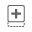When you hover over this button, the new argument placement is highlighted. When you click this button, a new empty item is created and opened in editing mode.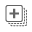Available for percentage function only - When you click this button, a submenu containing the following two choices are displayed. When you hover over one of these 2 choices, the placement of the new list of arguments is highlighted.

• Add all items from the whole form adds all suitable form items from all form sections.
• Add all items above within the section adds suitable form items only from the same section the calculate field belongs to, and which are positioned above it.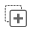When you hover over this button, the placement of the new parent item is highlighted. When you click this button, a new default item is added between the selected item and it’s current parent. The new parent item is opened in editing mode so you can select which function/operation you want the new parent item to be.

## Delete #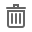When you click this button for a form item or numeric value it is simply deleted. When you click this button for a function or operation, a submenu containing the following two choices are displayed.

• Only this function performs a deletion of only the function/operation itself. In that case, all descendants are  preserved and become the children of the deleted item's parent.
• Whole function subtree deletes an item with the whole subtree (with all descendants). When you hover over one of these two choices, the items to be deleted are highlighted.

## Expression vs tree items #

When you hover over the item inside an expression, the corresponding item is highlighted inside the builder tree and vice versa. When you click the item inside the expression the corresponding item is switched to editing mode inside the builder tree.

## Error handling #

Some functions and operations can take only a specific type of parameters or a specific number of parameters. So, when you somehow break these rules, you get an error message. This message is placed immediately above the item label (or in the case of an empty value or unknown form component instead of its label)  inside the builder tree. When your expression contains any error you cannot click the Update button to save your changes until corrected.

## Number logic #

The Calculate component can use the same number logic as described in the Number component.